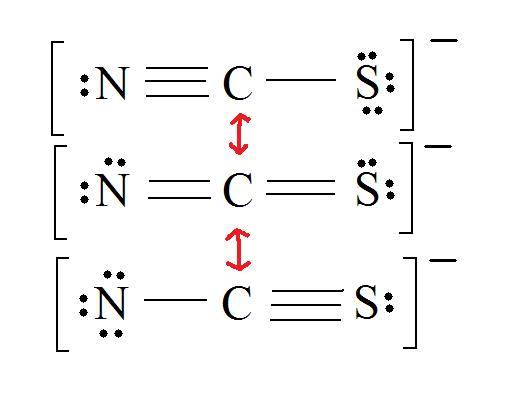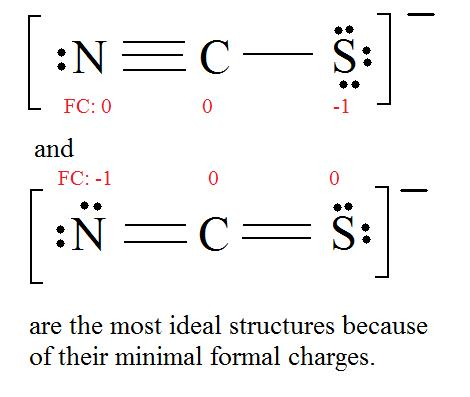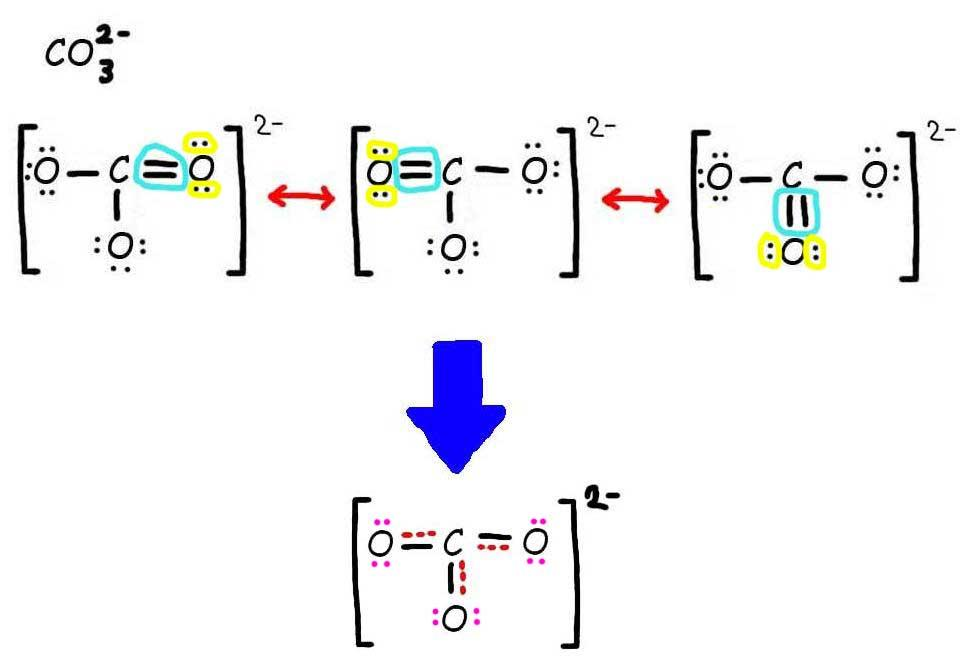# 10.4 Stability of the Allyl Radical: Resonance Revisited

Objectives

After completing this section, you should be able to

1. explain the stability of the allyl radical in terms of resonance.
2. explain the difference between resonance and tautomerism.
3. write an equation for the reaction of an unsymmetrical alkene with N-bromosuccinimide.
4. draw the structure of each of the possible products that could be obtained from the reaction of a given unsymmetrical alkene with N-bromosuccinimide, and predict which product will predominate.
5. explain the formation of more than one product from the reaction of N-bromosuccinimide with a given unsymmetrical alkene.
6. explain the observed product ratio when a given unsymmetrical alkene is treated with N-bromosuccinimide.

Key Terms

Make certain that you can define, and use in context, the key terms below.

• delocalized
• resonance forms
• resonance hybrid

Study Notes

You will have encountered the concept of resonance if you have taken general first-year chemistry course. You should also briefly review Section 2.5.

When we can represent a species by two or more different Lewis or Kekulé structures, neither of which represents the true structure of the species, these structures are referred to as resonance forms. A common example used in general chemistry courses to illustrate the concept of resonance is ozone, O3. The two resonance forms of ozone may be represented as follows:The concept of resonance is quite important, and will be used frequently throughout the remainder of this course. The guidelines below may assist you in drawing resonance contributors.

1. Resonance occurs whenever a molecule, radical or ion can be represented by two or more structures differing only in the arrangement of electrons (no atoms may be moved).
2. The true structure of a species is a hybrid of the resonance contributors and is more stable (i.e., lower in energy) than any of the contributors.
3. The most important contributors are those containing the most covalent bonds. Another way of saying the same thing is that the most important contributors have the least amount of charge separation.
4. Contributors in which all the atoms (except hydrogen) have a complete octet (i.e., are surrounded by eight electrons) are particularly important.

In the previous section we discussed the allylic bromination of a symmetrical alkene with NBS such as this cyclopentene, which affords one product.However, with an unsymmetrical alkene and the delocalized unpaired electron forming various allylic resonances, several products are possible. For example, the NBS bromination of 4-methyl-cyclohexene leads to three products.## The geometry and relative stability of carbon radicals

As organic chemists, we are particularly interested in radical intermediates in which the unpaired electron resides on a carbon atom. Experimental evidence indicates that the three bonds in a carbon radical have trigonal planar geometry, and therefore the carbon is considered to be sp2-hybridized with the unpaired electron occupying the perpendicular, unhybridized 2pzorbital. Contrast this picture with carbocation and carbanion intermediates, which are both also trigonal planar but whose 2pz orbitals contain zero or two electrons, respectively.The trend in the stability of carbon radicals parallels that of carbocations (section 8.4B): tertiary radicals, for example, are more stable than secondary radicals, followed by primary and methyl radicals. This should make intuitive sense, because radicals, like carbocations, can be considered to be electron deficient, and thus are stabilized by the electron-donating effects of nearby alkyl groups. Benzylic and allylic radicals are more stable than alkyl radicals due to resonance effects - an unpaired electron can be delocalized over a system of conjugated pi bonds. An allylic radical, for example, can be pictured as a system of three parallel 2pz orbitals sharing three electrons.## Introduction

Electrons have no fixed position in atoms, compounds and molecules (see image below) but have probabilities of being found in certain spaces (orbitals). Resonance forms illustrate areas of higher probabilities (electron densities). This is like holding your hat in either your right hand or your left. The term Resonance is applied when there are two or more possibilities available. Resonance structures do not change the relative positions of the atoms like your arms in the metaphor. The skeleton of the Lewis Structure remains the same, only the electron locations change. A double headed arrow on both ends of the arrow ( $$\leftrightarrow$$ ) between Lewis structures is used to show their inter-connectivity. It is different from the double harpoons ( $$\rightleftharpoons$$ ) used for designating equilibria. A double headed arrow on only one end ( $$\rightarrow$$ ) is used to indicate the movement of two electrons in a single resonance structure.

Example 10.4.1

Consider ozone (O3)

SOLUTIONFigure: This is an animation of how one can do a resonance with ozone by moving electrons:

## Delocalization and Resonance Structures Rules

In resonance structures, the electrons are able to move to help stabilize the molecule. This movement of the electrons is called delocalization.

1. Resonance structures should have the same number of electrons, do not add or subtract any electrons. (You can check the number of electrons by counting them)
2. All resonance structures must follow the rules of writing Lewis Structures.
3. The hybridization of the structure must stay the same.
4. The skeleton of the structure can not be changed (only the electrons move).
5. Resonance structures must also have the same amount of lone pairs.

## Formal Charge

Even though the structures look the same, the formal charge (FC) may not be. Formal charges are charges that are assigned to a specific atom in a molecule. If computed correctly, the overall formal charge of the molecule should be the same as the oxidation charge of the molecule (the charge when you write out the empirical and molecular formula) We want to choose the resonance structure with the least formal charges that add up to zero or the charge of the overall molecule.

The equation for finding Formal Charge is:

Formal Charge = (number of valence electrons in free orbital) - (number of lone-pair electrons) - ( $$\frac{1}{2}$$ number bond pair electrons)

The formal charge has to equal the molecule's overall charge.

Ex.) CNS- has an overall charge of -1, so the Lewis structure's formal charge has to equal -1.

Example 10.4.2: Thiocyanate Ion

Consider the thiocyanate (CNS-) ion.

SOLUTION

1. Find the Lewis Structure of the molecule. (Remember the Lewis Structure rules.)2. Resonance: All elements want an octet, and we can do that in multiple ways by moving the terminal atom's electrons around (bonds too).3. Assign Formal Charges

Formal Charge = (number of valence electrons in free orbital) - (number of lone-pair electrons) - ( $$\frac{1}{2}$$ number bond pair electrons)

Remember to determine the number of valence electron each atom has before assigning Formal Charges

C = 4 valence e-, N = 5 valence e-, S = 6 valence e-, also add an extra electron for the (-1) charge. The total of valence electrons is 16.4. Find the most ideal resonance structure. (Note: It is the one with the least formal charges that adds up to zero or to the molecule's overall charge.)5. Now we have to look at electronegativity for the "Correct" Lewis structure.

The most electronegative atom usually has the negative formal charge, while the least electronegative atom usually has the positive formal charges.## Resonance Hybrids

Resonance Structures are a representation of a Resonance Hybrid, which is the combination of all resonance structures. Though the Formal Charge closest to zero is the most accepted structure, in reality the correct Lewis structure is actually a combination of all the resonance structures (and hence is not solely describe as one).

1. Draw the Lewis Structure & Resonance for the molecule (using solid lines for bonds).
2. Where there can be a double or triple bond, draw a dotted line (-----) for a bond.
3. Draw only the lone pairs found in all resonance structures, do not include the lone pairs that are not on all of the resonance structures.

Example 10.4.3

Consider the carbonate ion: CO32-

SOLUTIONStep 1: Draw the Lewis Structure & Resonance.Step 2: Combine the resonance structures by adding (dotted) bonds where other resonance bonds can be formed.Step 3: Add only the lone pairs found on ALL resonance structures.The bottom is the finished resonance hybrid for CO32-.

## Exercises

#### Questions

Q10.4.1

The following reaction shows the major product. Explain why this would be the final product and why the 2° bromo product is not the major product.Q10.4.2

Predict the products of the following reactions:#### Solutions

S10.4.1

The product (A) is a 1° halogen which is more predominant product even though the (B) had a better transition state with a 2° radical. The 1° radical intermediate is not as sterically hindered.S10.4.2